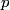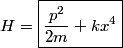## Solution to 1986 Problem 35

 The Hamiltonian in this case is just the total energy as a function of$p$ and$x$.\begin{align*}H = \boxed{\frac{p^2}{2m} + k x^4}\end{align*}Therefore, answer (A) is correct.